## Green's Function--Poisson's Equation

Poisson's Equation equation is(1)

whereis often called a potential function anda density function, so the differential operator in this case is. As usual, we are looking for a Green's function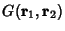such that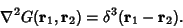(2)

But from Laplacian,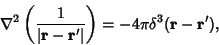(3)

so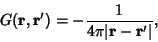(4)

and the solution is(5)

Expandingin the Spherical Harmonics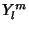gives(6)

where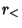andare Greater Than/Less Than Symbols. This expression simplifies to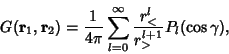(7)

whereare Legendre Polynomials, and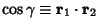. Equations (6) and (7) give the addition theorem for Legendre Polynomials.

In Cylindrical Coordinates, the Green's function is much more complicated,(8)

whereand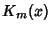are Modified Bessel Functions of the First and Second Kinds (Arfken 1985).

References

Arfken, G. Mathematical Methods for Physicists, 3rd ed. Orlando, FL: Academic Press, pp. 485-486, 905, and 912, 1985.